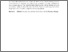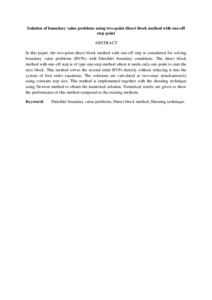# Solution of boundary value problems using two-point direct block method with one-off step point

## Citation

Zakaria, Nurul Nadirah and Abdul Majid, Zanariah and Lee, Lai Soon (2017) Solution of boundary value problems using two-point direct block method with one-off step point. Far East Journal of Mathematical Sciences, 101 (2). 375 - 394. ISSN 0972-0871

## Abstract

In this paper, the two-point direct block method with one-off step is considered for solving boundary value problems (BVPs) with Dirichlet boundary conditions. The direct block method with one-off step is of type one-step method where it needs only one-point to start the next block. This method solves the second order BVPs directly without reducing it into the system of first order equations. The solutions are calculated at two-steps simultaneously using constant step size. This method is implemented together with the shooting technique using Newton method to obtain the numerical solution. Numerical results are given to show the performance of this method compared to the existing methods.Preview
Text (Abstract)
Solution of boundary value problems using two-point direct block method with one-off .pdfView Item